### Tolerance Distribution

For a single independent variable, such as a dosage level, the models for the probabilities can be justified on the basis of a population with mean
and scale parameterof tolerances for the subjects. Then, given a dose x, the probability, P, of observing a response in a particular subject is the probability that the subject’s tolerance is less than the dose or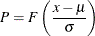Thus, in this case, the intercept parameter,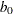, and the regression parameter,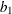, are related toandby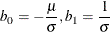Note: The parameteris not equal to the standard deviation of the population of tolerances for the logistic and extreme value distributions.